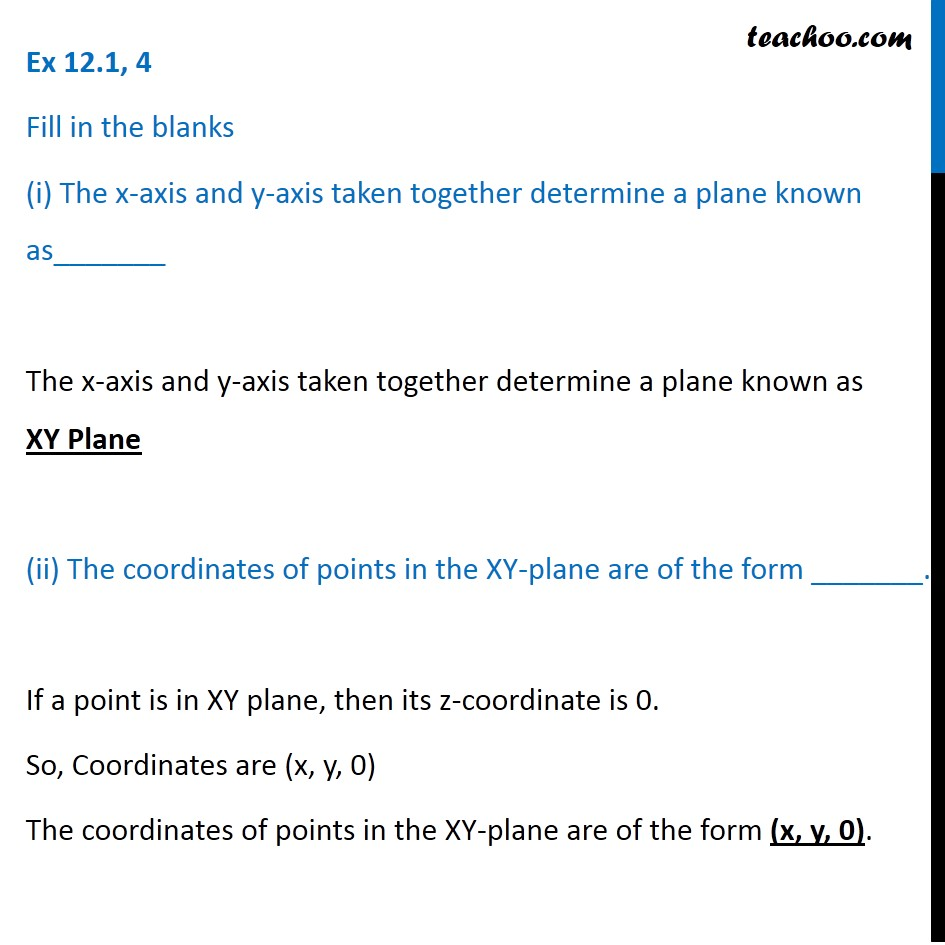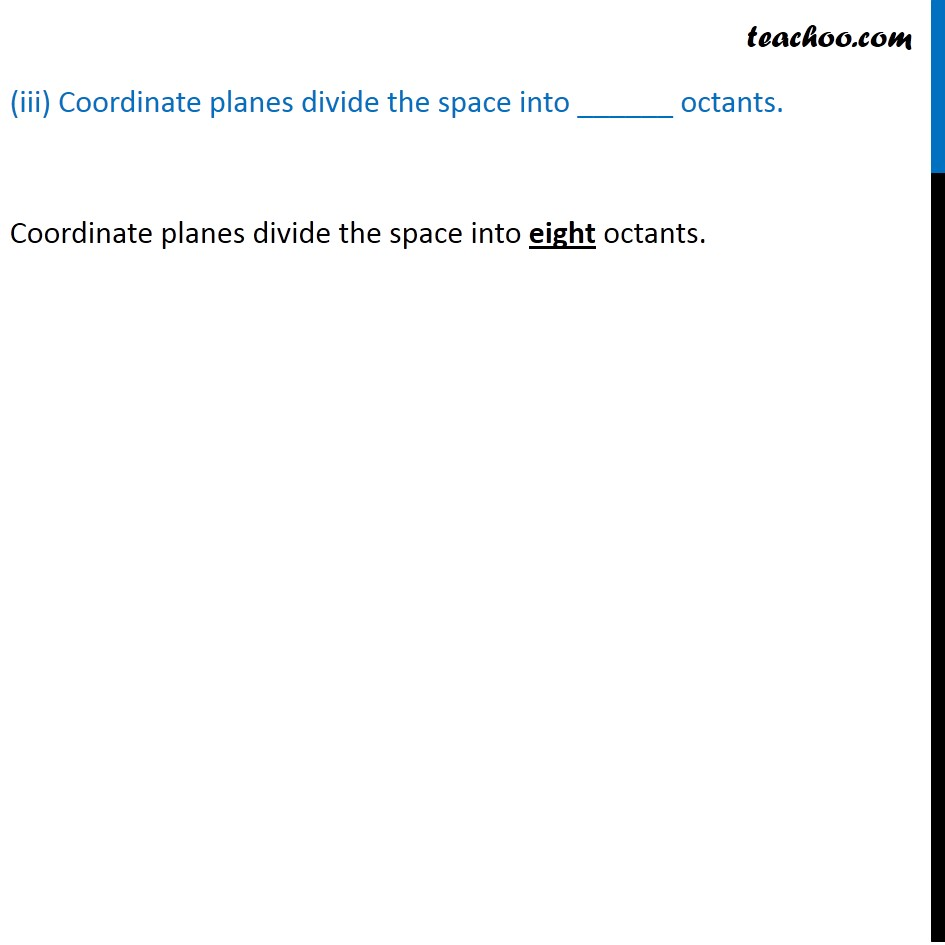Ex 11.1

Chapter 11 Class 11 - Intro to Three Dimensional Geometry
Serial order wiseLearn in your speed, with individual attention - Teachoo Maths 1-on-1 Class

### Transcript

Ex 11.1, 4 Fill in the blanks (i) The x-axis and y-axis taken together determine a plane known as_______ The x-axis and y-axis taken together determine a plane known as XY Plane (ii) The coordinates of points in the XY-plane are of the form _______. If a point is in XY plane, then its z-coordinate is 0. So, Coordinates are (x, y, 0) The coordinates of points in the XY-plane are of the form (x, y, 0). (iii) Coordinate planes divide the space into ______ octants. Coordinate planes divide the space into eight octants.